Next: Perturbed planetary orbit Up: Derivation of Gauss planetary Previous: Derivation of Gauss planetary

# Unperturbed planetary orbit

Consider a planet (say) of massand relative position vector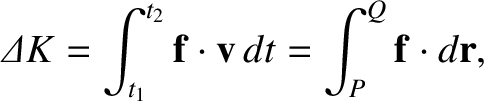that is orbiting around the Sun, whose mass is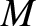. The planet's unperturbed equation of motion is written (see Section 4.16)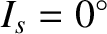(I.1)

where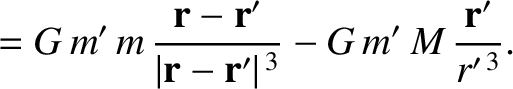. As described in Chapter 4, the solution to this equation is a Keplerian ellipse.

Let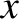,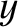,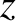be a Cartesian coordinate system in a reference frame whose origin corresponds to the location of the Sun, and which is such that the planet's unperturbed orbit lies in the plane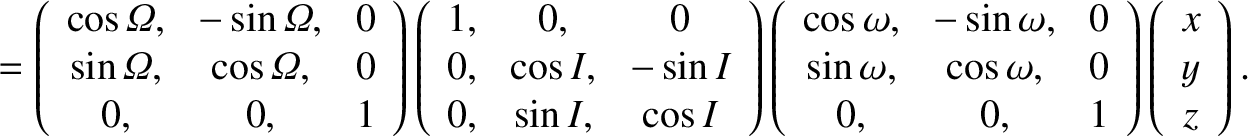, with the angular momentum vector pointing in the positive-direction, and the perihelion situated on the positive-axis. Let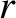,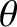,be a cylindrical coordinate system in the same reference frame.

We know from the analysis of Chapter 4 that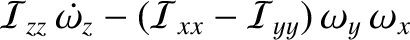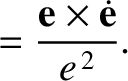(I.2) and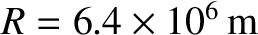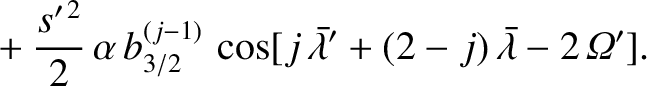(I.3)

where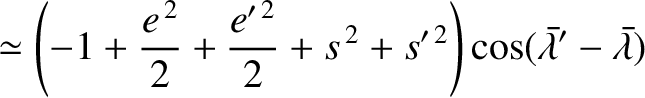and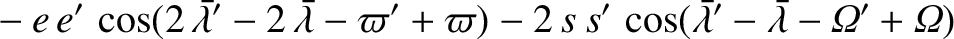. Moreover, the planet's mean orbital angular velocity is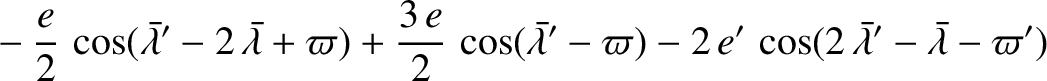(I.4)

its orbital energy per unit mass is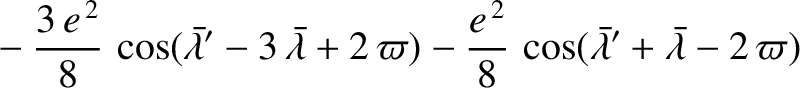(I.5)

its orbital angular momentum per unit mass is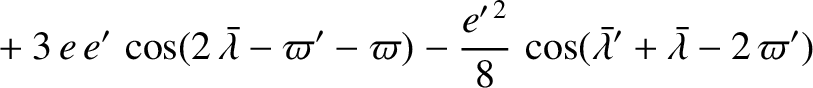(I.6)

where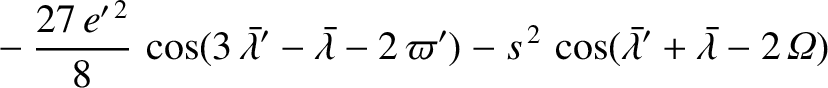(I.7)

and its eccentricity vector is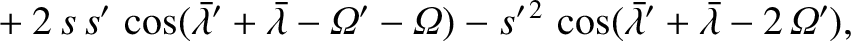(I.8)

Here,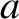and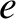are the planet's orbital major radius and eccentricity, respectively. Note that, for the unperturbed orbit, the quantities,,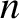,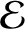,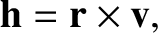, and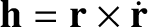are all constant in time. We also have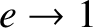(I.9)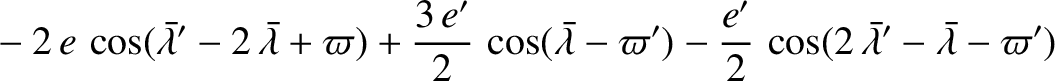(I.10)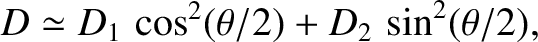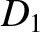(I.11)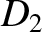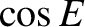(I.12) and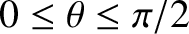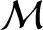(I.13)

where,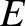, and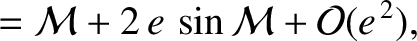are the planet's true anomaly, eccentric anomaly, and mean anomaly, respectively. (See Chapter 4.)Next: Perturbed planetary orbit Up: Derivation of Gauss planetary Previous: Derivation of Gauss planetary
Richard Fitzpatrick 2016-03-31